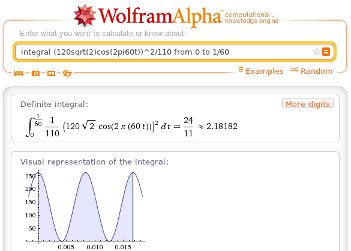# Numeric Integration tricks (Shallow Thoughts)

Akkana's Musings on Open Source Computing and Technology, Science, and Nature.

## Numeric Integration tricks

The MITx 6.002 "Circuits and Electronics" class started a week ago Monday. Exciting -- I'm hoping I'll be able to learn all those electronics concepts that baffle me while I'm trying to design simple circuits. (Assuming I make it -- I'm struggling and it's only the first week.)

One of the early exercises required integrating a trig function. No problem -- I used Wikipedia's tables of integrals. But subsequent discussion of that problem in the forums reminded me that when you're after a numeric solution, we do have computers to do that sort of thing for us.In particular, someone linked to Wolfram Alpha: `integral (120sqrt(2)cos(2pi60t))^2/110 from 0 to 1/60` A nifty tool that I should remember to use more often! Not only does it give you the numeric answer, but it also gives you a nice symbolic display (so you can make sure you typed in what you thought you were typing in), and a graph.

There was one hitch, though. In this particular problem, there was some debate over the integration limits -- should it be 0 to 1/60 or 0 to 1? If you try the same thing with 0 to 1, you still get the numeric answer, but you get "Computation timed out", with a link labeled "Try again with more time" that leads to an exhortation to subscribe to Wolfram Alpha Pro.

Of course, that made me antsy and made me wonder ... aren't there local solutions? What if I want to calculate an integral when I'm away from a fast network? Is there some way to do this using Python, Octave or R?

And of course there is. I haven't found an easy way to get the pretty graphics, but you can get the numerical results fairly easily.

In Octave, it's a bit roundabout: you have to define a function, then call quad. The easiest way is to use inline:

```octave:2> f = inline("(120 * sqrt(2) * cos(120 * pi * x))^2 / 110");
ans =  2.1818
```
though you can also define a function this way:
```octave:4> function y = ff(t)
>   y = (120 * sqrt(2) * cos(120 * pi * tx))^2 / 110;
> endfunction
ans =  2.1818
```

Here's how to do the same thing in Python using SciPy:

```import scipy
import math

scipy.integrate.quad(lambda t: (120 * math.sqrt(2) * math.cos(120 * math.pi * t))**2 / 110., 0, 1./60) * 60
```

Of course, you don't need to use lambda -- you can also define a function and pass it to scipy.integrate.quad.

None of this gets the nice graphics of Wolfram Alpha, though of course either Python or Octave can be programmed to generate them. I saw a presentation at PyCon about a package called Sage that can probably do nice graphics. But it's about a 350Mb download, so trying it wasn't an option during the conference, and now the site is down due to an electrical problem. So for now, Wolfram Alpha wins the graphics war.

Tags:
[ 20:44 Mar 13, 2012    More science | permalink to this entry | ]